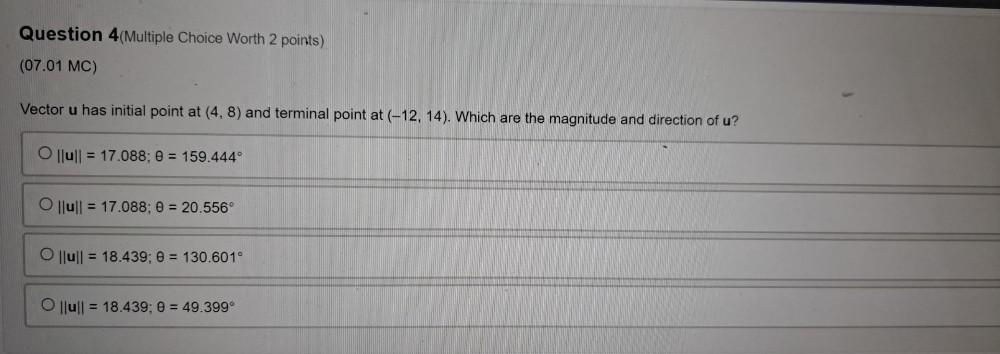Question:

# Vector u has initial point at (4,8) and terminal point at (-12, 14). Which are the magnitude and direction of u? a)lull = 17.088; θ = 159.444° b)|lull = 17.088; θ = 20.556° c)llull = 18.439; θ = 130.6Vector u has initial point at (4,8) and terminal point at (-12, 14). Which are the magnitude and direction of u? a)lull = 17.088; θ = 159.444° b)|lull = 17.088; θ = 20.556° c)llull = 18.439; θ = 130.601° d)|lull = 18.439; θ = 49.399